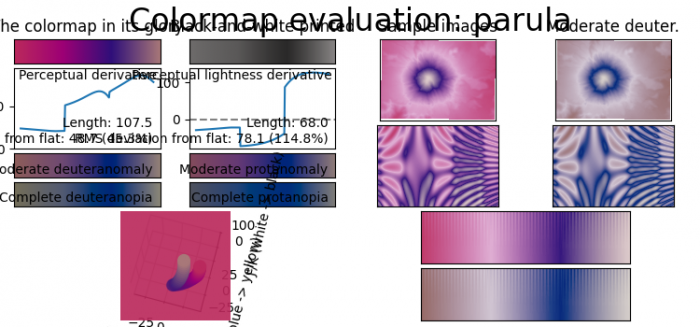# How to make the Parula colormap in Matplotlib?

To make the Parula colormap in matplotlib, we can take the following steps

• Set the figure size and adjust the padding between and around the subplots.
• Create colormap data using numpy.
• Create a 'LinearSegmentedColormap' from a list of colors.
• Viscum is a little tool for analyzing colormaps and creating new colormaps.
• Use imshow() method to display data as an image, i.e., on a 2D regular raster.
• To display the figure, use show() method.

## Example

from matplotlib.colors import LinearSegmentedColormap
import matplotlib.pyplot as plt
import numpy as np
from viscm import viscm
plt.rcParams["figure.figsize"] = [7.50, 3.50]
plt.rcParams["figure.autolayout"] = True
cm_data = np.random.rand(4, 4)
parula_map = LinearSegmentedColormap.from_list('parula', cm_data)
viscm(parula_map)
plt.imshow(np.linspace(0, 100, 256)[None, :], aspect='auto', cmap=parula_map)
plt.show()

## Output